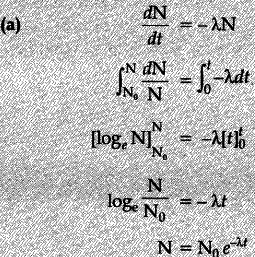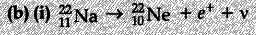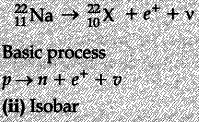# Deduce the expression

(a) Deduce the expression, $N={ N }{ 0 }{ e }^{ -\lambda t },$ for the law of radioactive decay.
b) (i) Write symbolically the process expressing the ${ \beta }^{ + }decay\quad of { 11 }^{ 22 }$ Na. Also write the basic nuclear
process underlying this decay.
(ii) Is the nucleus formed in the decay of the nucleus $_{ 11 }^{ 22 }$ Na, an isotope or an isobar ?Also accept, if a student does not identify the product nucleus and writes as